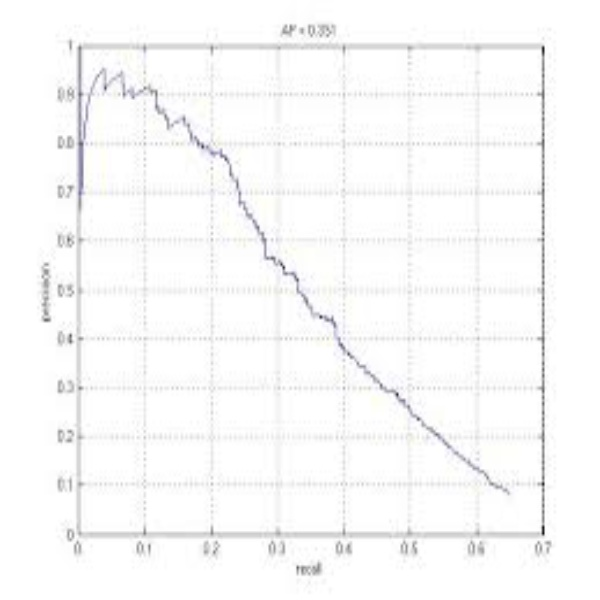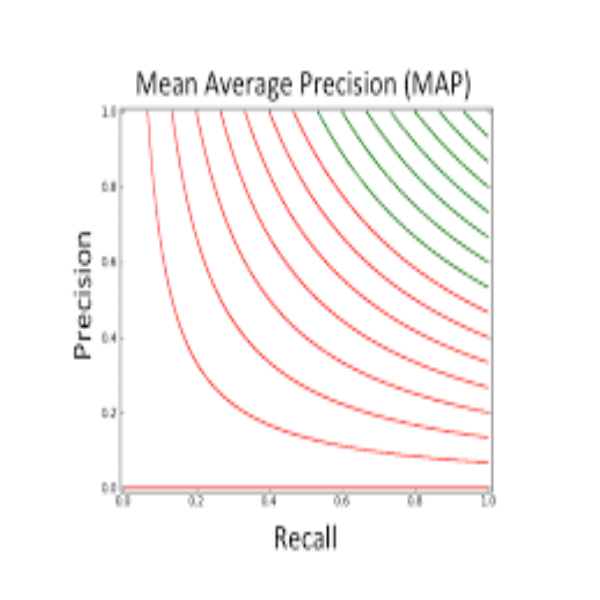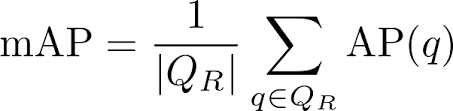# 深度学习: mAP (Mean Average Precision)

## mAP 概念

precision，即 准确率

recall，即 召回率

### PR曲线### AP值

Average Precision，即 平均精确度

### mAP值

Mean Average Precision，即 平均AP值## mAP 计算

### 公式### Code

def compute_ap(gt_boxes, gt_class_ids,
pred_boxes, pred_class_ids, pred_scores,
iou_threshold=0.5):
"""Compute Average Precision at a set IoU threshold (default 0.5).

Returns:
mAP: Mean Average Precision
precisions: List of precisions at different class score thresholds.
recalls: List of recall values at different class score thresholds.
overlaps: [pred_boxes, gt_boxes] IoU overlaps.
"""
# Trim zero padding and sort predictions by score from high to low
gt_boxes = trim_zeros(gt_boxes)
pred_boxes = trim_zeros(pred_boxes)
pred_scores = pred_scores[:pred_boxes.shape]
indices = np.argsort(pred_scores)[::-1]
pred_boxes = pred_boxes[indices]
pred_class_ids = pred_class_ids[indices]
pred_scores = pred_scores[indices]

# Compute IoU overlaps [pred_boxes, gt_boxes]
overlaps = compute_overlaps(pred_boxes, gt_boxes)

# Loop through ground truth boxes and find matching predictions
match_count = 0
pred_match = np.zeros([pred_boxes.shape])
gt_match = np.zeros([gt_boxes.shape])
for i in range(len(pred_boxes)):
# Find best matching ground truth box
sorted_ixs = np.argsort(overlaps[i])[::-1]
for j in sorted_ixs:
# If ground truth box is already matched, go to next one
if gt_match[j] == 1:
continue
# If we reach IoU smaller than the threshold, end the loop
iou = overlaps[i, j]
if iou < iou_threshold:
break
# Do we have a match?
if pred_class_ids[i] == gt_class_ids[j]:
match_count += 1
gt_match[j] = 1
pred_match[i] = 1
break

# Compute precision and recall at each prediction box step
precisions = np.cumsum(pred_match) / (np.arange(len(pred_match)) + 1)
recalls = np.cumsum(pred_match).astype(np.float32) / len(gt_match)

# Pad with start and end values to simplify the math
precisions = np.concatenate([, precisions, ])
recalls = np.concatenate([, recalls, ])

# Ensure precision values decrease but don't increase. This way, the
# precision value at each recall threshold is the maximum it can be
# for all following recall thresholds, as specified by the VOC paper.
for i in range(len(precisions) - 2, -1, -1):
precisions[i] = np.maximum(precisions[i], precisions[i + 1])

# Compute mean AP over recall range
indices = np.where(recalls[:-1] != recalls[1:]) + 1
mAP = np.sum((recalls[indices] - recalls[indices - 1]) *
precisions[indices])

return mAP, precisions, recalls, overlaps